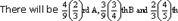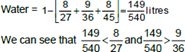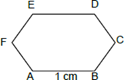# CAT 2017 – Slot 2 – Quantitative Ability – If a, b, c are three positive integers such that a and b are in the ratio 3 : 4

Q. 1: If a, b, c are three positive integers such that a and b are in the ratio 3 : 4 while b and c are in the ratio 2:1, then which one of the following is a possible value of (a + b + c)?
A) 201
B) 205
C) 207
D) 210

Explanation:- a : b = 3 : 4 and b : c = 2 : 1 Multiplying the second ratio by 2, we have

a : b = 3 : 4 b : c = 4 : 2

Therefore, a : b : c = 3 : 4 : 2.

⇒ a = 3k, b = 4k, c = 2k.

Since a, b and c are positive integers, their sum should be 9k, a positive integer. From the options, only choice (3), which is 207, is a multiple of 9.

##### Q. 2: A motorbike leaves point A at 1 pm and moves towards point B at a uniform speed. A car leaves point B at 2 pm and moves towards point A at a uniform speed which is double that of the motorbike. They meet at 3:40 pm at a point which is 168 km away from A. What is the distance, in km, between A and B?A) 364 B) 378 C) 380 D) 388

Explanation:- The motorbike that left A travelled 168 km from 1:00 p.m. to 3:40 p.m., i.e. in 2 2/3 hours or 8/3 hours. The car that left B, started at 2:00 p.m. and travelled till 3:40 p.m., i.e. for 1 2/3 hours or 5/3 hours.

The car would’ve travelled 5/8th of the distance as the bike, but since the car travelled at twice the speed, the car would’ve travelled 10/8th of the distance, i.e. (10/8) x 168 = 210 km.

Therefore, total distance between A and B = 168 + 210 = 378 km.

Q. 3: Amal can complete a job in 10 days and Bimal can complete it in 8 days. Amal, Bimal and Kamal together complete the job in 4 days and are paid a total amount of Rs 1000 as remuneration. If this amount is shared by them in proportion to their work, then Kamal’s share, in rupees, is
A) 100
B) 200
C) 300
D) 400

Explanation:- The team completes the job in 4 days.

Since Amol needs 10 days to complete the job, in 4 days, he does 4/10th or 40% of the work. Since Bimal needs 8 days to complete the job, in 4 days, he does 4/8th or 50% of the work.

Therefore, Kamal did 10% of the work and for doing 10% of the work, he gets 10% of the payment, i.e. Rs. 100.

Q. 4: Consider three mixtures – the first having water and liquid A in the ratio 1 : 2, the second having water and liquid B in the ratio  1 : 3, and the third having water and liquid C in the ratio 1 : 4. These three mixtures of A, B, and C, respectively, are further  mixed in the proportion 4:3:2. Then the resulting mixture has
A) The same amount of water and liquid B
B) The same amount of liquids B and C
C) More water than liquid B
D) More water than liquid A

Explanation:- First mixture has 2/3rds A and the rest water. Second mixture has 3/4ths B and the rest water. Third mixture has 4/5ths C and the rest water.

The 3 mixtures are mixed in the ratio 4 : 3 : 2. Let’s say we get a 1 litre mixture containing the three mixtures.C. (the remaining part would be water) 8/27 litres A, 9/36 litres B, 8/45 litres C∴ There is more water than B in the resultant solution. Choice (3)

Q. 5: Let ABCDEF be a regular hexagon with each side of length 1 cm. The area (in sq cm) of a square with AC as one side is
A) 3√2
B) 3
C) 4
D) √3Explanation:-

In ∆ABC, AB = 1 cm, BC = 1 cm As ∠B = 120,

AC2 = 12 + 12 – 2(1) (1) cos (120°) (Cosine rule)

= 12 + 12 + 1 = 3

⇒ AC = √3 cm

The square of side √3 cm will have an area of (√3 )2 = 3 cm2

##### Checkout Other Questions of CAT 2017 Slot 2 Paper:

Verbal Ability :              |   Q.01- Q.06  |  Q.07- Q.12  |  Q.13- Q.18  |  Q.19- Q.21  |  Q.22- Q.24  |  Q.25- Q.29  |  Q.30 – Q.34  |

Logical Reasoning :    |   Q.29 – Q.32  |

Quantitative Aptitude: |

### Free Material Area